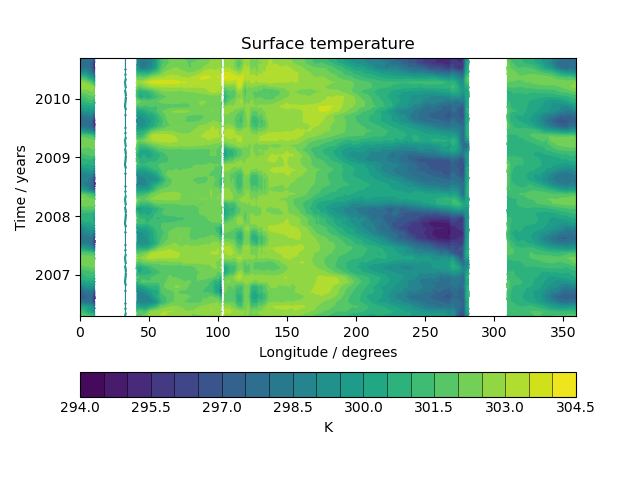# Hovmoller Diagram of Monthly Surface Temperature#

This example demonstrates the creation of a Hovmoller diagram with fine control over plot ticks and labels. The data comes from the Met Office OSTIA project and has been pre-processed to calculate the monthly mean sea surface temperature.```import matplotlib.dates as mdates
import matplotlib.pyplot as plt

import iris
import iris.plot as iplt
import iris.quickplot as qplt

def main():
# load a single cube of surface temperature between +/- 5 latitude
fname = iris.sample_data_path("ostia_monthly.nc")
fname,
iris.Constraint("surface_temperature", latitude=lambda v: -5 < v < 5),
)

# Take the mean over latitude
cube = cube.collapsed("latitude", iris.analysis.MEAN)

# Now that we have our data in a nice way, lets create the plot
# contour with 20 levels
qplt.contourf(cube, 20)

# Put a custom label on the y axis
plt.ylabel("Time / years")

# Stop matplotlib providing clever axes range padding
plt.axis("tight")

# As we are plotting annual variability, put years as the y ticks
plt.gca().yaxis.set_major_locator(mdates.YearLocator())

# And format the ticks to just show the year
plt.gca().yaxis.set_major_formatter(mdates.DateFormatter("%Y"))

iplt.show()

if __name__ == "__main__":
main()
```

Total running time of the script: ( 0 minutes 0.296 seconds)

Gallery generated by Sphinx-Gallery